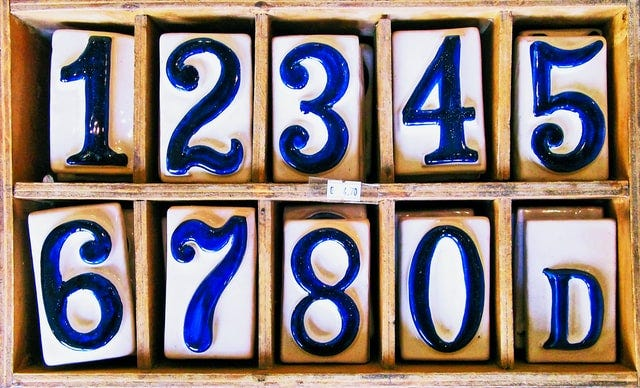# Python: Using the range() functionPhoto by Andrew Buchanan on Unsplash

This is a summary of Chapter 4 of “Python Crash Course: A Hands-On, Project Based Introduction to Programming” by Eric Matthes

# Using the range() Function

The range() function allows us to generate a series of numbers. For example:

`for i in range(0, 3):    print(i)>>> 0>>> 1>>> 2`

As you can see from the above code, the code will print the numbers from 0 (inclusive) up until 3 (exclusive). This is the off-by-one behavior you will see a lot in many programming languages. In Python, the range() function starts the count at the first value you give it, and it stops at the second value you provide while excluding that second value.

If you wanted to print out the numbers from one to three, you would use range(1, 4):

`for i in range(1, 4):    print(i)>>> 1>>> 2>>> 3`

# Using range() to make a List of Numbers

Wrap the list() function around a call to the range() function and the output will be a list of numbers. For example:

`numbers = list(range(0, 4))print(numbers)>>> [0, 1, 2, 3]`

If you wanted to skip numbers in a given range, all you need to do is add a step. For example:

`even_numbers = list(range(0, 30, 2))print(even_numbers)>>> [0, 2, 4, 6, 8, 10, 12, 14, 16, 18, 20, 22, 24, 26, 28]`

You can create almost any set of numbers using the range() function. One example is that you can make a list of squared numbers.

`squared = []for value in range(1, 4):    square = value ** 2    squared.append(square)print(squared)>>> [1, 4, 9]`

We first create an empty list called squared. Then we tell Python to loop through each value from one to four using the range() function. Inside the loop, the current value will be raised to the second power and that value will then be stored into the square variable. The last line in the for loop will append each new value of square into the list of squared. At the end of the code, the list of squared values will be printed.

# Simple Statistics with a List of Numbers

`numbers = [0, 1, 2, 3]print(min(numbers))print(max(numbers))print(sum(numbers))>>> 0 #min>>> 3 #max>>> 6 #sum`

The min() function gives us the minimum of the entire list of numbers.

The max() function gives us the maximum of the entire list of numbers.

The sum() function calculates the added total of the entire list of numbers.

# List Comprehensions

A list comprehension allows us to generate a list in just one line of code. They usually combine the for loop and the creation of new elements into one line and automatically appends each new item. For example:

`squared = [value ** 2 for value in range(1, 4)]print(squared)>>> [1, 4, 9]`

We begin by writing a descriptive name for the list, in this case, we used squared. Then we open a set of square brackets and define the expression for the values you would like to store into the new list. In this case, the expression is value ** 2. Something important to notice is that no colon is used at the end of the for statement. The result is the same as the earlier examples.

Using list comprehensions is useful when you’re writing three or four lines of code to generate lists since they usually begin feeling repetitive. It does take time and practice, but they will eventually be fast and efficient to use once you get the hang of them.

## Probably Programming

I’m explaining what I know to myself.## Probably Programming

I’m explaining what I know to myself.

Medium is an open platform where 170 million readers come to find insightful and dynamic thinking. Here, expert and undiscovered voices alike dive into the heart of any topic and bring new ideas to the surface. Learn more

Follow the writers, publications, and topics that matter to you, and you’ll see them on your homepage and in your inbox. Explore

If you have a story to tell, knowledge to share, or a perspective to offer — welcome home. It’s easy and free to post your thinking on any topic. Write on Medium